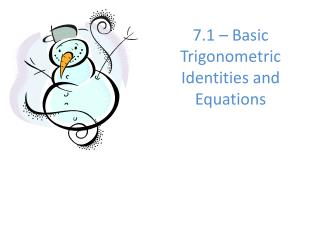Download Presentation7.1 – Basic Trigonometric Identities and Equations

7.1 – Basic Trigonometric Identities and Equations - PowerPoint PPT Presentation

7.1 – Basic Trigonometric Identities and Equations. Trigonometric Identities. Quotient Identities. Reciprocal Identities. Pythagorean Identities. sin 2 q + cos 2 q = 1. tan 2 q + 1 = sec 2 q. cot 2 q + 1 = csc 2 q. sin 2 q = 1 - cos 2 q. tan 2 q = sec 2 q - 1.I am the owner, or an agent authorized to act on behalf of the owner, of the copyrighted work described.
Download Presentation7.1 – Basic Trigonometric Identities and Equations

Download Policy: Content on the Website is provided to you AS IS for your information and personal use and may not be sold / licensed / shared on other websites without getting consent from its author.While downloading, if for some reason you are not able to download a presentation, the publisher may have deleted the file from their server.

- - - - - - - - - - - - - - - - - - - - - - - - - - E N D - - - - - - - - - - - - - - - - - - - - - - - - - -
Presentation Transcript
1. 7.1 – Basic Trigonometric Identities and Equations

2. Trigonometric Identities Quotient Identities Reciprocal Identities Pythagorean Identities sin2q + cos2q = 1 tan2q + 1 = sec2q cot2q + 1 = csc2q sin2q = 1 - cos2q tan2q = sec2q - 1 cot2q = csc2q - 1 cos2q = 1 - sin2q 5.4.3

3. Where did our pythagorean identities come from?? Do you remember the Unit Circle? • What is the equation for the unit circle? x2 + y2 = 1 • What does x = ? What does y = ? • (in terms of trig functions) sin2θ + cos2θ = 1 Pythagorean Identity!

4. Take the Pythagorean Identity and discover a new one! Hint: Try dividing everything by cos2θ sin2θ + cos2θ = 1 . cos2θcos2θ cos2θ tan2θ + 1 = sec2θ Quotient Identity Reciprocal Identity another Pythagorean Identity

5. Take the Pythagorean Identity and discover a new one! Hint: Try dividing everything by sin2θ sin2θ + cos2θ = 1 . sin2θsin2θ sin2θ 1 + cot2θ = csc2θ Quotient Identity Reciprocal Identity a third Pythagorean Identity

6. Using the identities you now know, find the trig value. 1.) If cosθ = 3/4, find secθ 2.) If cosθ = 3/5, find cscθ.

7. 3.) sinθ = -1/3, find tanθ 4.) secθ = -7/5,find sinθ

8. REMEMBER…. TO NUMBER EACH STEP WRITE CLEARLY GO ALL THE WAY TO ONE TRIG VALUE (DON’T LEAVE TAN2X, LEAVE TANX)

9. Simplifying Trigonometric Expressions Identities can be used to simplify trigonometric expressions. Simplify. b) a) 5.4.5

10. Simplifing Trigonometric Expressions c) (1 + tan x)2 - 2 sin x sec x d)

11. Simplify each expression.

12. sin x cos x Simplifying trig Identity Example1: simplify tanxcosx tanx cosx tanxcosx = sin x

13. sec x sec x sin x sinx 1 1 1 = tan x = = x cos x cos x cos x csc x csc x sin x 1 Simplifying trig Identity Example2: simplify

14. cos2x - sin2x cos2x - sin2x cos2x - sin2x 1 = sec x cos x cos x Simplifying trig Identity Example2: simplify

15. Example Simplify: Factor out cot x = cot x (csc2 x - 1) Use pythagorean identity = cot x (cot2 x) Simplify = cot3 x

16. = 1 = sin2 x + cos2x = sin x (sin x) + cos x = sin2 x + (cos x) cos x cos x cos x cos x cos x cos x Example Simplify: Use quotient identity Simplify fraction with LCD Simplify numerator Use pythagorean identity Use reciprocal identity = sec x

17. Your Turn! Combine fraction Simplify the numerator Use pythagorean identity Use Reciprocal Identity

18. Practice

19. One way to use identities is to simplify expressions involving trigonometric functions. Often a good strategy for doing this is to write all trig functions in terms of sines and cosines and then simplify. Let’s see an example of this: substitute using each identity simplify

20. Another way to use identities is to write one function in terms of another function. Let’s see an example of this: This expression involves both sine and cosine. The Fundamental Identity makes a connection between sine and cosine so we can use that and solve for cosine squared and substitute.

21. (E) Examples • Prove tan(x) cos(x) = sin(x)

22. (E) Examples • Prove tan2(x) = sin2(x) cos-2(x)

23. (E) Examples • Prove

24. (E) Examples • Prove